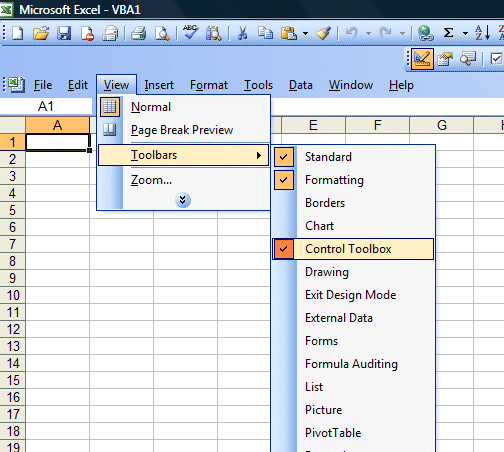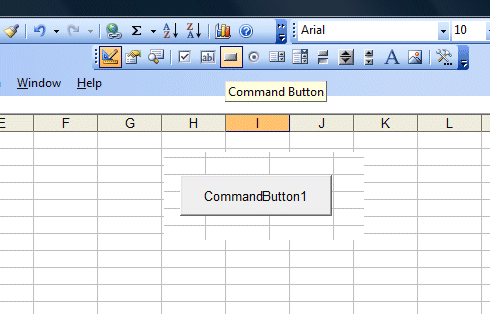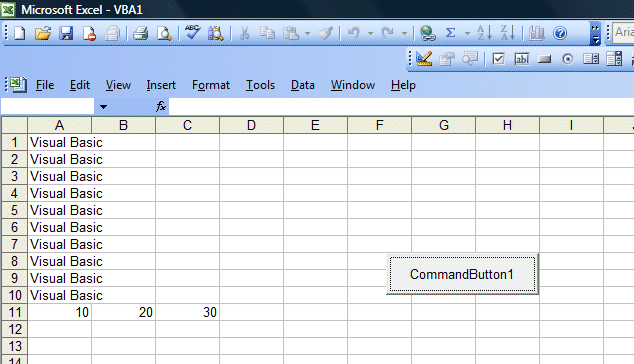Lesson 1: Introduction

Excel VBA stands for Excel Visual Basic for Applications. The application created using Excel VBA is also known as a macro. The macro can be created using the built-in Visual Basic Editor in Microsoft Excel. This tutorial is based on MS Excel 2003. If you wish to learn VBA for MS Excel 2010, please follow our Excel VBA 2010 Tutorial here. The macro syntaxes remain largely the same for newer versions of Microsoft Excel.

methods which you can create an Excel VBA macro. The first method is to place a command button on the MS Excel spreadsheet and click on the button to enter the Visual Basic Editor. The second method is to launch the Visual Basic Editor from the menu. To launch the VBE, click on tools on the menu bar and select macro and then click on Visual Basic Editor.

1.1 Creating Excel VBA Using the Command Button

To create an Excel VBA macro using the command button, you need to place it on the spreadsheet. In order to access the command button, you need to click View on the MS Excel menu bar and then click on the toolbar and select the Control Toolbox to launch the control toolbox, as shown in Figure 1.1. The control toolbox comprises various controls, as shown in Figure 1.2. Select the command button and place it on the spreadsheet.Figure 1.1>

Figure 1.2: The Control Toolbox

Next, click on the command button to start the Visual Basic Editor. In the VBE , enter the statements as shown in Figure 1.3. The first statement will fill up cell A1 to cell A10 with the phrase "Visual Basic" while the second statement adds the value in cell A11 and cell B11  and then displays the sum in cell C11. The output is shown in Figure 1.4.Figure 1.3 Visual Basic Editor1.2 Creating an Excel VBA Macro App in Visual Basic Editor

To launch the VBE, click on tools on the menu bar and select macro and then click on Visual Basic Editor, as shown in Figure 1.5. The Visual Basic Editor code window is shown in Figure 1.6. In this example, we create a VBA function using the If..Then..ElseIf statements. This function computes the commission based on the sales volume. We will learn more about function in the later lesson.Figure 1.5